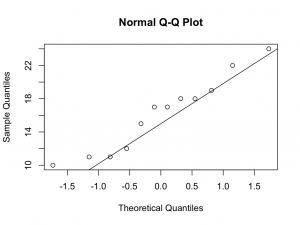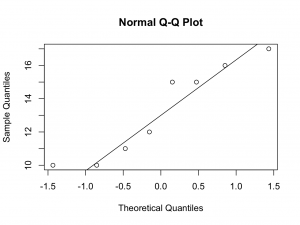1. Using <= 16 weeks as ‘cut off’ point, indicate patients were fracture healing had occurred within 16 weeks by ‘-‘ and patients who took longer than 16 weeks to unite by ‘+’. In the smoking group, there were 7 out of 12 patients were fracture union was in excess of 16 weeks. Whilst In the non smokers, there was only 1 out of 8 patients were the fracture took longer than 16 weeks to unite. Is this due to chance?We formulate a null hypothesis; there is no difference in fracture healing time between smokers and non smokers and test this hypothesis. Using a two sided sign test the probability is:P > 5%, therefore NOT statistically significant. The null hypothesis can therefore NOT be rejected. We conclude that we were unable to demonstrate a difference in fracture healing time between smokers and non smokers.

The same result is obtained if the calculation is performed in R / JGR:

binom.test(1,8)

Exact binomial test

data:  1 and 8
number of successes = 1, number of trials = 8, p-value = 0.07031
alternative hypothesis: true probability of success is not equal to 0.5
95 percent confidence interval:
0.003159724 0.526509671
sample estimates:
probability of success
0.125

1. Using the Chi Square test, we need to first construct a table with the observed frequencies and the expected frequencies:
Smoker
Observed
Smoker
Expected
Non Smoker
Observed
Non Smoker
Expected
Total
12128820
<= 16 weeks512x12/20 = 7.278x12/20 = 4.812
> 16 weeks712x8/20 = 4.818x8/20 = 3.28
Using the Chi Square test statistic:The observed frequency table has two rows and two columns. There is therefore 1 degree of freedom (r-1)×(c-1). Using the Chi Square distribution table we can see that there is statistical significance (p < 5%). However, not all prerequisites of the Chi Square test have been met as the expected frequencies are not above 5. It would therefore be more appropriate to use the Yates continuity correction (should be used if the expected frequencies are below 10) or the Fisher Exact test (if expected frequencies are below 5). These tests are performed below in R / JGR.

First without continuity correction:

data<-matrix(c(5,7,7,1),nrow=2)
chisq.test(data,correct=FALSE)

Pearson’s Chi-squared test

data:  data
X-squared = 4.2014, df = 1, p-value = 0.04039

Warning message:
In chisq.test(data, correct = FALSE) :
Chi-squared approximation may be incorrect

Obviously, R / JGR calculates the same result. The computer calculates an exact p-value, suggesting significance. However, the expected frequencies are below 5 and it is inappropriate to use the Chi Square test. To show the expected frequencies:

chisq.test(data,correct=FALSE)$expected [,1] [,2] [1,] 7.2 4.8 [2,] 4.8 3.2 Warning message: In chisq.test(data, correct = FALSE) : Chi-squared approximation may be incorrect If the expected frequencies are below 10, Yates continuity correction should be used: chisq.test(data,correct=TRUE) Pearson’s Chi-squared test with Yates’ continuity correction data: data X-squared = 2.5087, df = 1, p-value = 0.1132 Warning message: In chisq.test(data, correct = TRUE) : Chi-squared approximation may be incorrect So, the Chi Square test with Yates continuity correction is insignificant! If the expected frequencies are below 5 however, a Fisher Exact test would be more appropriate: fisher.test(data) Fisher’s Exact Test for Count Data data: data p-value = 0.06967 alternative hypothesis: true odds ratio is not equal to 1 95 percent confidence interval: 0.001993953 1.370866340 sample estimates: odds ratio 0.1148794 Again, the result is insignificant. 1. To peform a Wilcoxon test in R / JGR: wilcox.test(smokers$Time~smokers$Group,correct=FALSE) Wilcoxon rank sum test data: smokers$Time by smokers$Group W = 26.5, p-value = 0.0949 alternative hypothesis: true location shift is not equal to 0 Warning message: In wilcox.test.default(x = c(10L, 10L, 11L, 12L, 15L, 15L, 16L, : cannot compute exact p-value with ties The same result can be obtained by using the Deducer GUI. Select ‘Analysis’ and ‘Two Sample Test’. Select Time as outcome, Group as factor, tick ‘Wilcoxon’ and click ‘Run’. The Wilcoxon test is insignificant, again failing to demonstrate a difference. 1. Normality will be tested using three methods; a quantile quantile plot, a Shapiro-Wilk test and a Kolmogorov-Smirnov test. To create a quantile quantile plot for both groups: qqnorm(smokers$Time)
qqline(smokers$Time) qqnorm(smokers$Time[which(smokers$Group==’Smoker’)]) qqline(smokers$Time[which(smokers$Group==’Smoker’)])And for only the non smokers: qqnorm(smokers$Time[which(smokers$Group==’Non Smoker’)]) qqline(smokers$Time[which(smokers$Group==’Non Smoker’)])Although the numbers are small, the quantile quantile plots deviate from a straight line at the lower quantiles. Therefore, it may not be appropriate to assume Normality. The Shapiro-Wilk test can be performed on both groups together, or separately: shapiro.test(smokers$Time)

Shapiro-Wilk normality test

data:  smokers$Time W = 0.9233, p-value = 0.1148 shapiro.test(smokers$Time[which(smokers$Group==’Smoker’)]) Shapiro-Wilk normality test data: smokers$Time[which(smokers$Group == “Smoker”)] W = 0.9393, p-value = 0.4884 shapiro.test(smokers$Time[which(smokers$Group==’Non Smoker’)]) Shapiro-Wilk normality test data: smokers$Time[which(smokers$Group == “Non Smoker”)] W = 0.8837, p-value = 0.2041 The p-value is insignificant and there is no reason to reject the null hypothesis that the data is Normally distributed. It is concluded it would be reasonable to model the data with a Normal distribution. The Kolomogorov-Smirnov test can be used to compare the variable time to a Normal distribution with the same mean and standard deviation: ks.test(smokers$Time,”pnorm”,mean=mean(smokers$Time),sd=sd(smokers$Time))

One-sample Kolmogorov-Smirnov test

data:  smokers$Time D = 0.1683, p-value = 0.6226 alternative hypothesis: two-sided Warning message: In ks.test(smokers$Time, “pnorm”, mean = mean(smokers$Time), sd = sd(smokers$Time)) :
ties should not be present for the Kolmogorov-Smirnov test

Or, comparing both groups:

ks.test(smokers$Time[which(smokers$Group==’Smoker’)],smokers$Time[which(smokers$Group==’Non Smoker’)])

Two-sample Kolmogorov-Smirnov test

data:  smokers$Time[which(smokers$Group == “Smoker”)] and smokers$Time[which(smokers$Group == “Non Smoker”)]
D = 0.4583, p-value = 0.2656
alternative hypothesis: two-sided

Warning message:
In ks.test(smokers$Time[which(smokers$Group == “Smoker”)], smokers$Time[which(smokers$Group ==  :
cannot compute exact p-value with ties

Again, there is no reason to reject the null hypothesis and Normality is assumed on the basis of the Shapiro-Wilk and Kolmogorov-Smirnov tests.

The Kolmogorov-Smirnov test can also be performed using the Deducer GUI by selecting ‘Analysis’, Two Sample Tests’, Time as outcome, Group as factor and ticking the Kolmogorov-Smirnov box.

If a t-test is performed, the result is also insignificant (p=0.09).

t.test(smokers$Time~smokers$Group)

Welch Two Sample t-test

data:  smokers$Time by smokers$Group
t = -1.7848, df = 17.976, p-value = 0.09117
alternative hypothesis: true difference in means is not equal to 0
95 percent confidence interval:
-6.3501621  0.5168288
sample estimates:
mean in group Non Smoker     mean in group Smoker
13.25000                 16.16667

1. The answer to question 4 suggests it is reasonable to assume Normality and therefore to use a t-test. To perform a power analsysis in R / JGR:

The mean of the Time variable is:

mean(smokers$Time)  15 The standard deviation of the Time variable is: sd(smokers$Time)
 4.091069

These descriptives can also be obtained from the Deducer GUI by selecting ‘Analysis’, ‘Descriptives’ and the required functions.

Delta is 10% of the mean, so delta is 1.5. The significance level is 0.05 and the power 80% (0.08):

power.t.test(sd=4.09,delta=1.5,sig.level=0.05,power=0.8)

Two-sample t test power calculation

n = 117.6764
delta = 1.5
sd = 4.09
sig.level = 0.05
power = 0.8
alternative = two.sided

NOTE: n is number in *each* group

Therefore, 118 patients are required in each group (study size 236).

1. First a 2 × 2 table is constructed:
Truth
Positive
Truth
Negative

361450
Test
Positive
6410
Test
Negative
301040
True Positive: 6, False Positive: 4, False Negative: 30, True Negative: 10ppv = 60%, npv = 25%, Sensitivity ≈ 17%, Specificity ≈ 71%, Accuracy = 32%

Or in the R / JGR console:

library(epiR)
mat<-matrix(c(6,30,4,10),ncol=2)
mat
[,1] [,2]
[1,]    6    4
[2,]   30   10
epi.tests(mat)
Disease +    Disease –      Total
Test +            6            4         10
Test –           30           10         40
Total            36           14         50

Point estimates and 95 % CIs:
———————————————————
Apparent prevalence                    0.20 (0.10, 0.34)
True prevalence                        0.72 (0.58, 0.84)
Sensitivity                            0.17 (0.06, 0.33)
Specificity                            0.71 (0.42, 0.92)
Positive predictive value              0.60 (0.26, 0.88)
Negative predictive value              0.25 (0.13, 0.41)
Positive likelihood ratio              0.58 (0.19, 1.76)
Negative likelihood ratio              1.17 (0.81, 1.68)
———————————————————

1. No